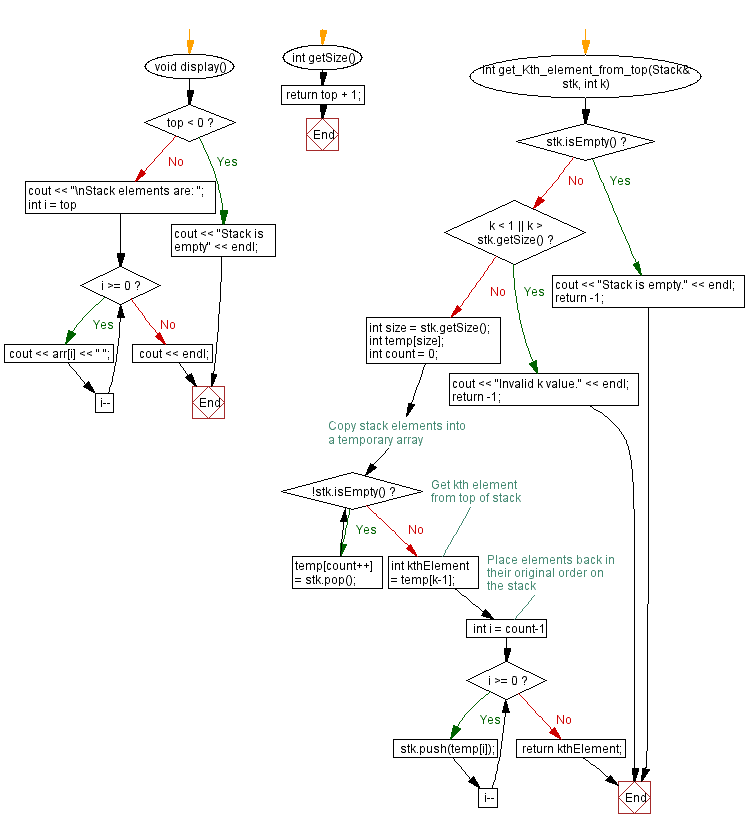﻿ C++ Kth element of a stack from top position

# C++ Stack Exercises: Kth element of a stack from top position

## C++ Stack: Exercise-14 with Solution

Write a C++ program to get the Kth element of a stack from top position.

Test Data:
Input some elements onto the stack:
Stack elements are: 4 3 2 1
Remove 2 from the said stack:
2nd element from the top of the stack: 3

Sample Solution:

C++ Code:

``````#include <iostream>

using namespace std;
#define MAX_SIZE 15 // Maximum size of stack
class Stack {
private:
int top; // Index of top element
int arr[MAX_SIZE]; // Array to store elements
public:
Stack() {
top = -1; // Initialize top index to -1 (empty stack)
}

bool push(int x) {
if (isFull()) {
cout << "Stack is full!" << endl;
return false;
}
// Increment top index and add element to array
arr[++top] = x;
return true;
}

int pop() {
if (isEmpty()) {
cout << "Stack underflow" << endl;
return 0;
}
return arr[top--];
}

int peek() {
if (isEmpty()) {
cout << "Stack is empty" << endl;
return 0;
}
return arr[top];
}

bool isEmpty() {
// Stack is empty if top index is -1
return (top < 0);
}

bool isFull() {
// Stack is full if top index is equal to MAX_SIZE - 1
return (top >= MAX_SIZE - 1);
}

void display() {
if (top < 0) {
cout << "Stack is empty" << endl;
return;
}
cout << "\nStack elements are: ";
for (int i = top; i >= 0; i--)
cout << arr[i] << " ";
cout << endl;
}
int getSize() {
}
int get_Kth_element_from_top(Stack& stk, int k) {
if (stk.isEmpty()) {
cout << "Stack is empty." << endl;
return -1;
}
if (k < 1 || k > stk.getSize()) {
cout << "Invalid k value." << endl;
return -1;
}
int size = stk.getSize();
int temp[size];
int count = 0;
// Copy stack elements into a temporary array
while (!stk.isEmpty()) {
temp[count++] = stk.pop();
}
// Get kth element from top of stack
int kthElement = temp[k-1];
// Place elements back in their original order on the stack
for (int i = count-1; i >= 0; i--) {
stk.push(temp[i]);
}
return kthElement;
}
};
int main() {
Stack stk;
cout << "Input some elements onto the stack:";
stk.push(1);
stk.push(2);
stk.push(3);
stk.push(4);
stk.display();
cout << "\nRemove 2 from the said stack:";
int z = stk.get_Kth_element_from_top(stk, 2);
cout << "\n2nd element from the top of the stack: " << z;
cout << "\n\nInput two more elements onto the stack:";
stk.push(-1);
stk.push(0);
stk.display();
z = stk.get_Kth_element_from_top(stk, 1);
cout << "\n1st element from the top of the stack: " << z;
z = stk.get_Kth_element_from_top(stk, 3);
cout << "\n3rd element from the top of the stack: " << z;
z = stk.get_Kth_element_from_top(stk, 6);
cout << "\n6th element from the top of the stack: " << z;
cout << endl;
}
``````

Sample Output:

```Input some elements onto the stack:
Stack elements are: 4 3 2 1

Remove 2 from the said stack:
2nd element from the top of the stack: 3

Input two more elements onto the stack:
Stack elements are: 0 -1 4 3 2 1

1st element from the top of the stack: 0
3rd element from the top of the stack: 4
6th element from the top of the stack: 1
```

Flowchart:CPP Code Editor: